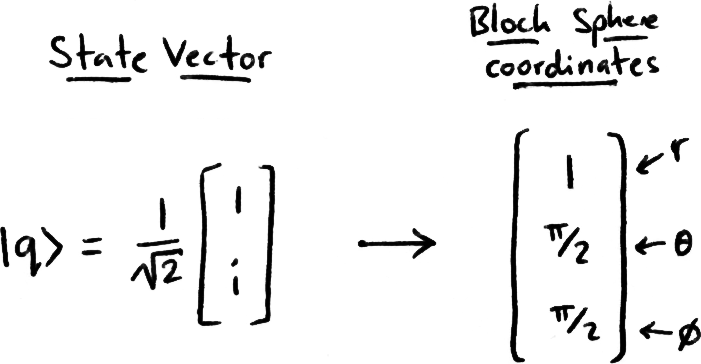# Quantum Computing

## The Qubit, Superposition & Measurement

Here we introduce the quantum mechanical concepts needed for quantum computing. We introduce some ‘postulates’ and look at what these statements imply about our quantum computer, it is hoped that covering quantum computing in this style will help students when covering general quantum mechanics.

## The Rules of Quantum Computing

There are certain statements in quantum mechanics we assume to be true without explanation (postulate), and the proof of their correctness comes from the accuracy of the predictions we derive from them. Here we give our own set of ‘postulates’, adapted from the postulates of quantum mechanics, and discuss their implications.

1. The state of a quantum register (collection of qubits) can be described as a vector |ψ⟩ in Hilbert space that contains all the information that can be known about the system.

Classically, the most simple system that can hold information is the bit. In quantum mechanics, the most simple system that can hold information is the qubit: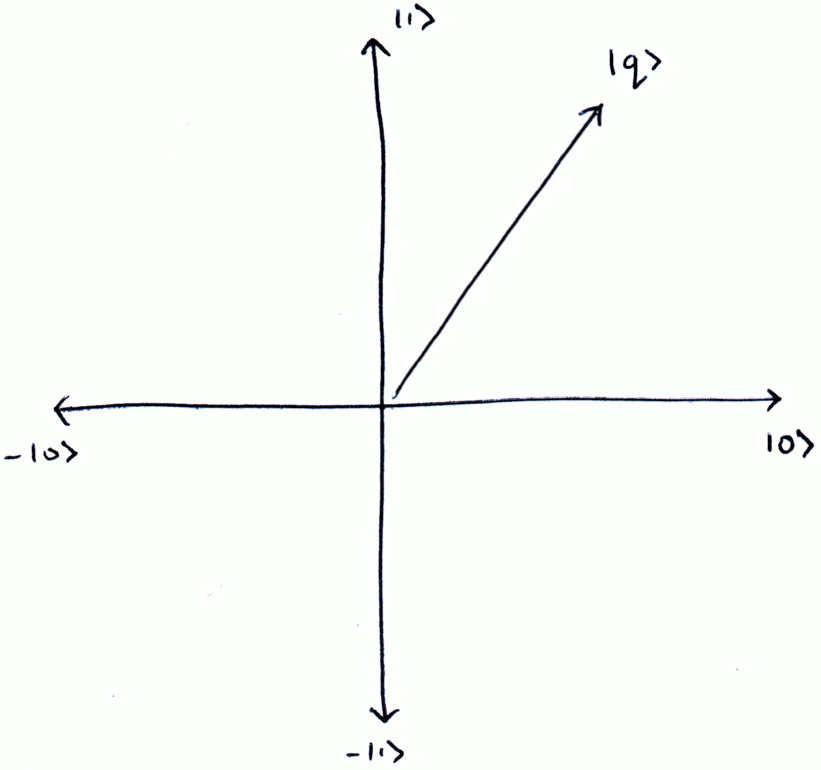As according to (1), the qubit can be described as a vector in Hilbert space, and the simplest vector we can have is a vector with two dimensions. Following the conventions of classical computing, we call these dimensions |0⟩ and |1⟩. This vector behaviour allows for our qubit to occupy the space spanned by |0⟩ & |1⟩, and we call this property superposition.

1. To measure a quantum register, we apply an Hermitian operator A
2. Upon measurement, the register will collapse into one of A's eigenvectors, with probability proportional to |a|2, where 'a' is the amplitude of that eigenvector.

There is a lot of information in this last statement, let's dissect it: Consider a single qubit, if our matrix A was: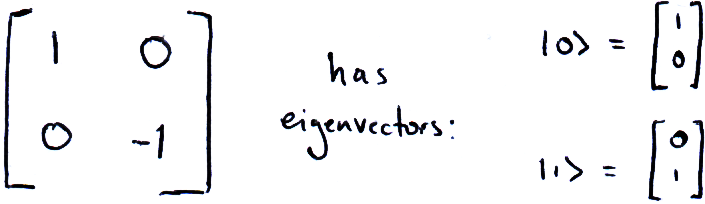Then our qubit |q⟩ = a|0⟩ + b|1⟩ would collapse to |0⟩ with probability |a|2 and |1⟩ with probability |b|2. By convention in quantum computing, we usually measure using in the matrix shown above. This collapses our qubits into either |0⟩ or |1⟩ and we call this measuring in the computational basis. This clearly tells us some more information about the behaviour of our qubit:

Firstly, since the probability of obtaining a measurement is 1, the magnitude of our qubit vector must always be normalised to 1.

Secondly, upon measurement, we destroy the superposition of our qubit and lose the information stored in its amplitudes. This is one of the unfortunate limitations of quantum computing: that despite all the information held in these qubit states we can only get a yes-or-no answer.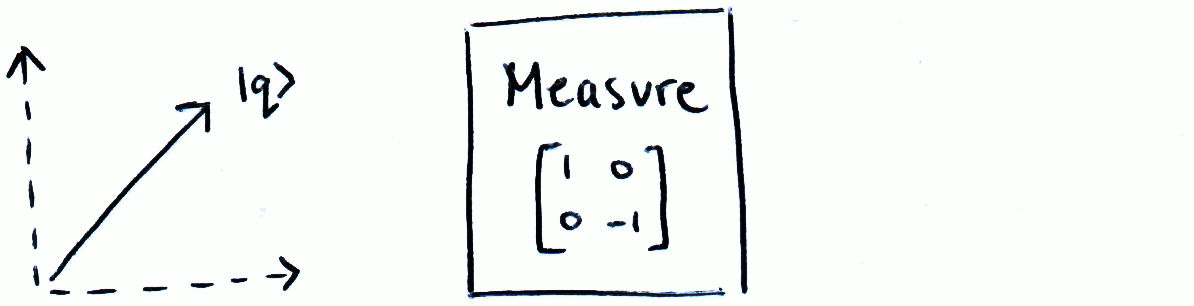## The Bloch Sphere

Since we square the magnitude of our amplitudes to find the probability of measurement, certain qubit states are equivalent to us. We can ignore global phase (phase applied to the whole qubit). For example: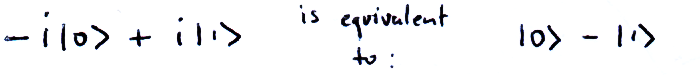We have narrowed down the possible states of our qubits; their squared amplitudes must equal 1 and we only care about the difference in phase between our two amplitudes (a & b). As a result, we can write the state of any qubit in the form: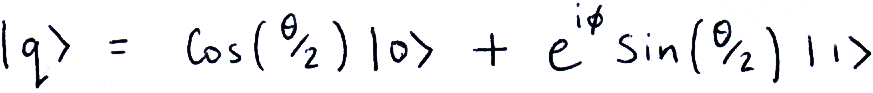Using the two variables θ and ϕ, we can represent each possible qubit state as a point on the surface of a sphere (the Bloch sphere):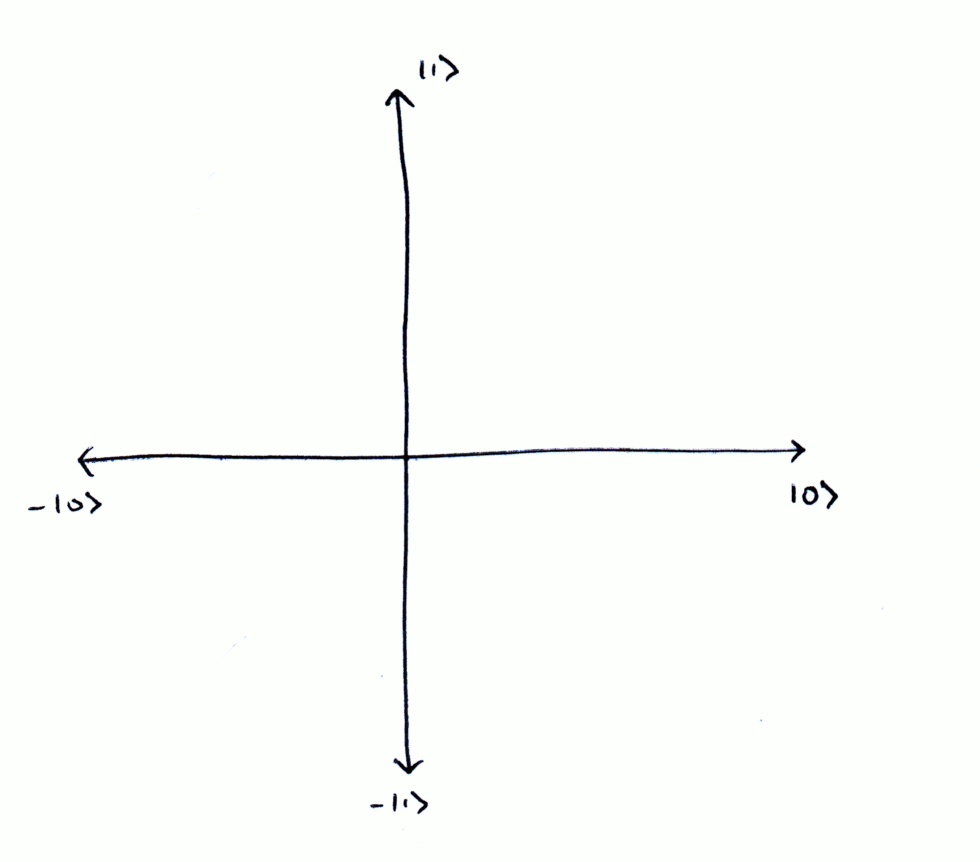The Bloch sphere is very useful for visualising single-qubit states and will help us understand the effects of single-qubit operations. Remember for a qubit state, it’s important not to confuse its coordinates on the Bloch sphere with its state vector!